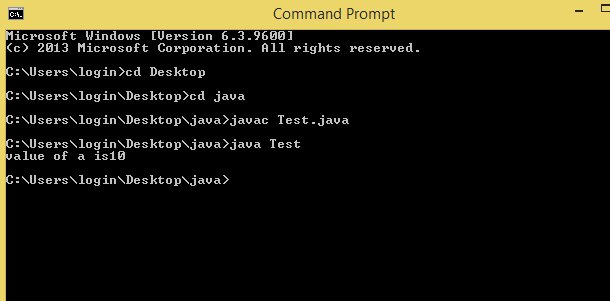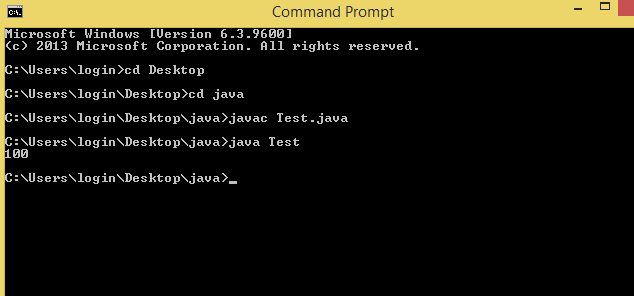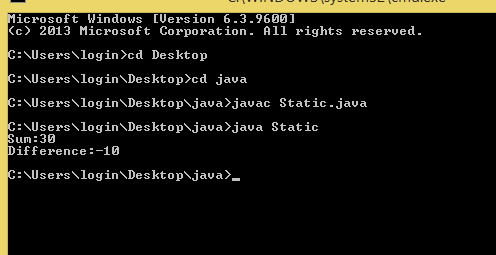×

# Variables?

A Java variable is a piece of memory that can contain a data value.A variable thus has a data type.Variables are typically used to store information which our Java program needs to do its task.

Syntax:data-type variable_name= value;
for eg: int a=10;
```
Variables Types:-
```

On basic of position and declaration variables are classified as:

1. Local variable
2. Instance variable
3. Static variable

4. ## Local variable:

1. A variable defined inside method or block is called a local variable.
2. A scope of these variable exists only within block in which the variable is declared i.e one can access the variable within that block or method only.
3. Initialization of variable is mendatory otherwise code is not executed.

Example:

class Test{

public static void main (String args[]){

//local variables

int a=10;

System.out.println("value of a is"+a);

}

}

Output:## Instance variable:

1. Variables which are declared in a class but not inside a particular method are called instance variables.
2. These variables are created when object of class is created.Initialization is not mendatory.
3. If these variables are not initialized then they return default values 0 or false.
4. Value of variable is varied from object to object.
5. These can't be accessed from static area directly,firstly we have to create object.
6. Example:

class Test{

//instance variable

int i=100;

public static void main(string args[]){

Abc a1=new Abc();

System.out.println(a1.i);

}

Output:## Static variable

1. Static variable also known as class variable.
2. These variable declared as same as instance variable but with static keyword within class.
3. Value is not varied from object to object.
4. They can access directly using class name.
5. Example:

class Static{

//static variable

static String sum="Sum:";

static String diff="Difference:";

public static void main(String[]args)

{

int a=10;

int b=20;

int c=a+b;

int d=a-b;

System.out.println(sum+c);

System.out.println(diff+d);

}

}

Output: